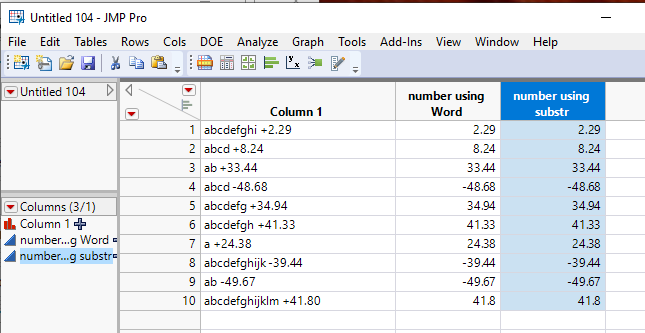Our World Statistics Day conversations have been a great reminder of how much statistics can inform our lives. Do you have an example of how statistics has made a difference in your life? Share your story with the Community!
Choose Language Hide Translation Bar
Highlighted

## Extract numbers from strings of unequal sizes

I have a column of strings with embedded numbers. I know how to determine if these strings contains the correct text I am looking for, but not how to extract the values that come after each of these. If the string sizes were equal, I could just use the munger function based on the location, but this cannot be done since string size is not consistent.

E.g "The fix in is abcde +0.2, there is more text here fghij -1."

I want to query for "abcde" and "fghij" and extract the +0.2 and -1 respective values in the space right after the strings appear. There is usually a space followed by a +/- and a number (whole numbers and decimals out to one decimal place as shown above).

3 REPLIES 3
Highlighted

## Re: Extract numbers from strings of unequal sizes

Check out the Word() function.  Given the examples you gave, one could simply look for the the 2nd word in the string, based upon finding a blank

Word(2, <your string column>, " " )

or

You can Contains() to find a "+" or "-" and from there, substr() the string to get the numeric value

Attached is the below data tableJim
Highlighted

## Re: Extract numbers from strings of unequal sizes

You can also use the Regex() function with regular expressions in a column formula. That approach might help if the example you gave does not show all the possible conditions. This is a script to illustrate the idea.

``````Names Default to Here( 1 );

text = "The fix in is abcde +0.2, there is more text here fghij -1.";

answer 1 = Num( Regex( text, ".*abcde ([-+]?\d?.?\d?)", "\1" ) );

answer 2 = Num( Regex( text, ".*fghij ([-+]?\d?.?\d?)", "\1" ) );``````

The text variable in the script represents the current row for a column formula. The answer 1 would be the result in the same row of the first new result column. You use the expression to the right of the equal sign for the formula.

This example is simple. It would need to be extended and refined if the nature of the text or the preface to the numbers were more complicated.

You also have JMP Patterns for matching, returning, and substituting character strings.

Learn it once, use it forever!
Highlighted

## Re: Extract numbers from strings of unequal sizes

Yet another way - try the words function.  This is the column formula:

``````look_for = {"abcde", "fghij", "klmno"};
wlist = Words( :Column 1, " " );
one_num = .;
For( i = 1, i <= N Items( look_for ), i++,
one_word = look_for[i];
f = Contains( wlist, one_word );
If( f,
one_num = Num( wlist[f + 1] );
Break();
);
);
one_num;``````
Article Labels

There are no labels assigned to this post.